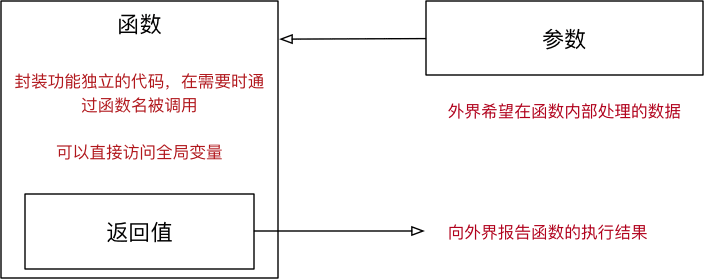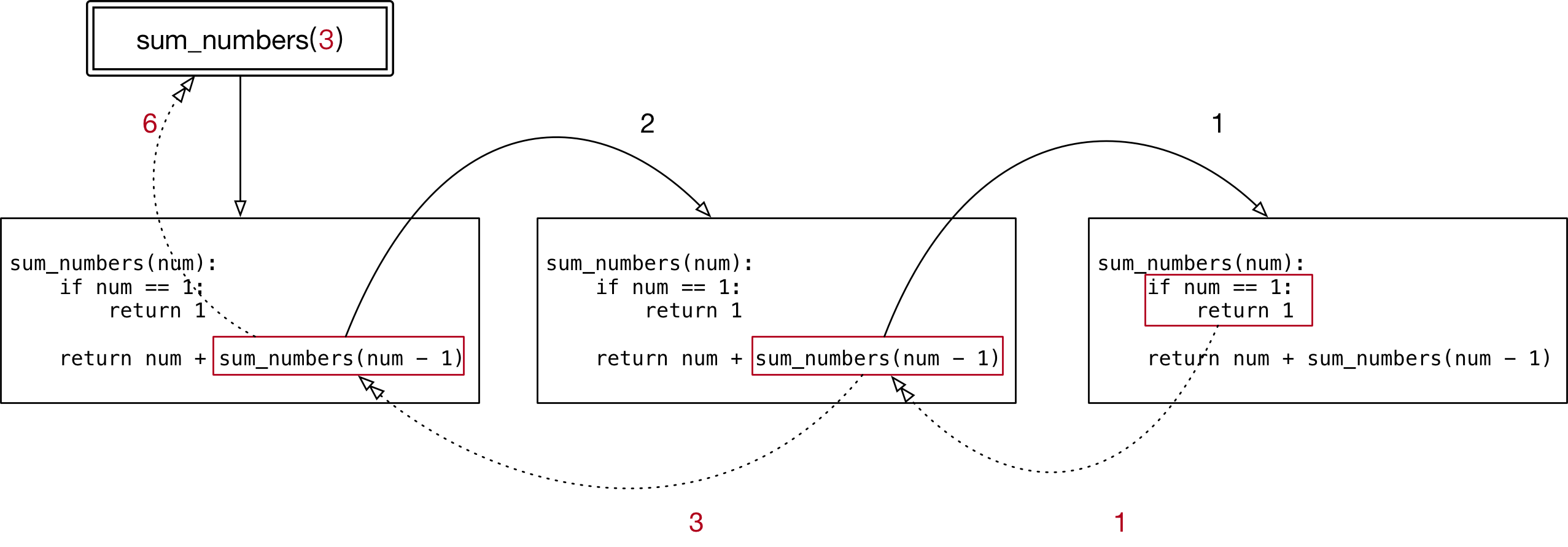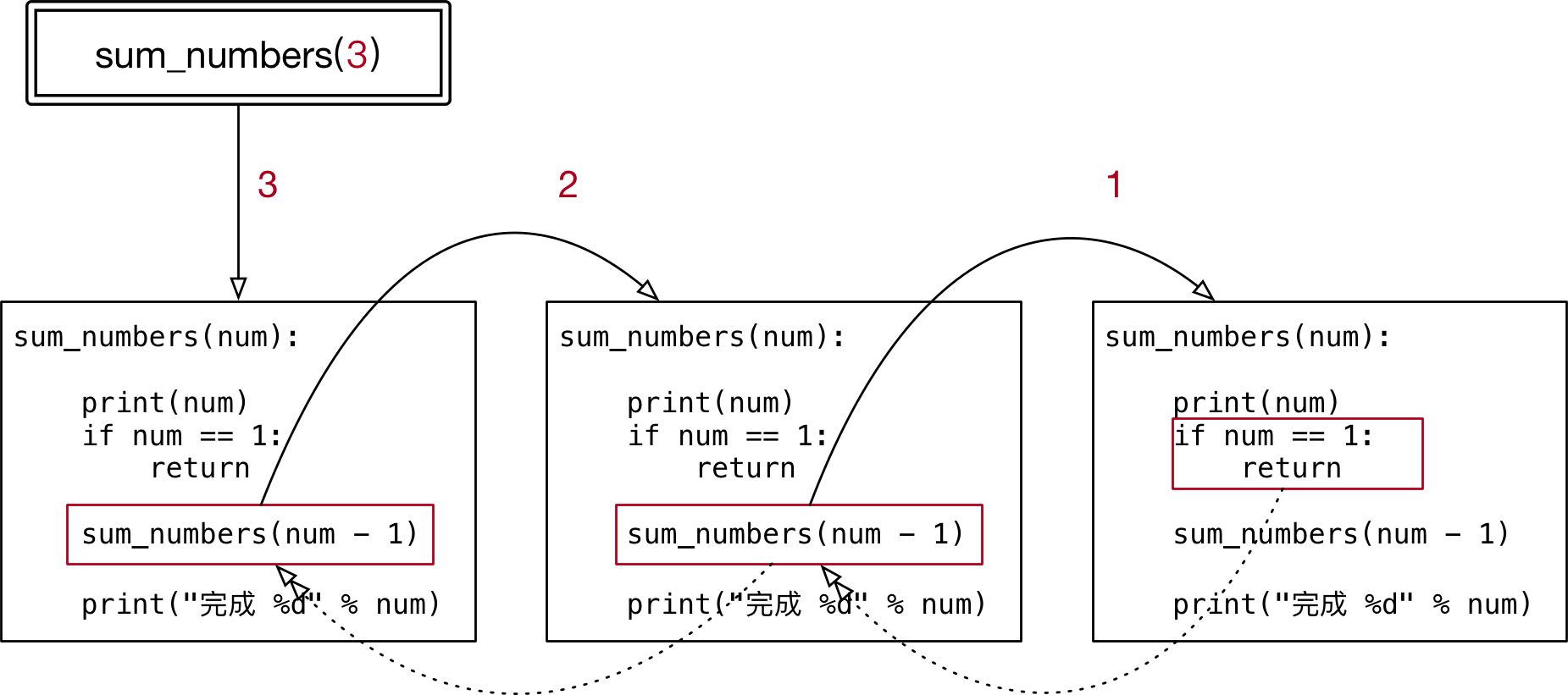• 函数的返回值 进阶
• 函数的参数 进阶
• 递归函数

# 01. 函数参数和返回值的作用

1. 无参数，无返回值
2. 无参数，有返回值
3. 有参数，无返回值
4. 有参数，有返回值定义函数时，是否接收参数，或者是否返回结果，是根据 实际的功能需求 来决定的！

1. 如果函数 内部处理的数据不确定，就可以将外界的数据以参数传递到函数内部
2. 如果希望一个函数 执行完成后，向外界汇报执行结果，就可以增加函数的返回值

## 1.1 无参数，无返回值

1. 只是单纯地做一件事情，例如 显示菜单
2. 在函数内部 针对全局变量进行操作，例如：新建名片，最终结果 记录在全局变量 中

• 如果全局变量的数据类型是一个 可变类型，在函数内部可以使用 方法 修改全局变量的内容 —— 变量的引用不会改变
• 在函数内部，使用赋值语句 才会 修改变量的引用

## 1.2 无参数，有返回值

• 采集数据，例如 温度计，返回结果就是当前的温度，而不需要传递任何的参数

## 1.3 有参数，无返回值

• 函数内部的代码保持不变，针对 不同的参数 处理 不同的数据
• 例如 名片管理系统 针对 找到的名片 做 修改、删除 操作

## 1.4 有参数，有返回值

• 函数内部的代码保持不变，针对 不同的参数 处理 不同的数据，并且 返回期望的处理结果
• 例如 名片管理系统 使用 字典默认值 和 提示信息 提示用户输入内容
• 如果输入，返回输入内容
• 如果没有输入，返回字典默认值

# 02. 函数的返回值 进阶

• 返回值 是函数 完成工作后，最后 给调用者的 一个结果
• 在函数中使用 return 关键字可以返回结果
• 调用函数一方，可以 使用变量 来 接收 函数的返回结果

• 假设要开发一个函数能够同时返回当前的温度和湿度
• 先完成返回温度的功能如下：
``````
def measure():
"""返回当前的温度"""

print("开始测量...")
temp = 39
print("测量结束...")

return temp

result = measure()
print(result)
``````
• 在利用 元组 在返回温度的同时，也能够返回 湿度
• 改造如下：
``````
def measure():
"""返回当前的温度"""

print("开始测量...")
temp = 39
wetness = 10
print("测量结束...")

return (temp, wetness)
``````

• 在 Python 中，可以 将一个元组 使用 赋值语句 同时赋值给 多个变量
• 注意：变量的数量需要和元组中的元素数量保持一致
``````
result = temp, wetness = measure()
``````

## 面试题 —— 交换两个数字

1. 有两个整数变量 a = 6, b = 100
2. 不使用其他变量，交换两个变量的值

``````
# 解法 1 - 使用临时变量
c = b
b = a
a = c
``````

``````
# 解法 2 - 不使用临时变量
a = a + b
b = a - b
a = a - b
``````

``````
a, b = b, a
``````

# 03. 函数的参数 进阶

## 3.1. 不可变和可变的参数

• 无论传递的参数是 可变 还是 不可变
• 只要 针对参数 使用 赋值语句，会在 函数内部 修改 局部变量的引用，不会影响到 外部变量的引用
``````
def demo(num, num_list):

print("函数内部")

# 赋值语句
num = 200
num_list = [1, 2, 3]

print(num)
print(num_list)

print("函数代码完成")

gl_num = 99
gl_list = [4, 5, 6]
demo(gl_num, gl_list)
print(gl_num)
print(gl_list)

``````

``````
def mutable(num_list):

# num_list = [1, 2, 3]
num_list.extend([1, 2, 3])

print(num_list)

gl_list = [6, 7, 8]
mutable(gl_list)
print(gl_list)
``````

• 在 python 中，列表变量调用 += 本质上是在执行列表变量的 extend 方法，不会修改变量的引用
``````
def demo(num, num_list):

print("函数内部代码")

# num = num + num
num += num
# num_list.extend(num_list) 由于是调用方法，所以不会修改变量的引用
# 函数执行结束后，外部数据同样会发生变化
num_list += num_list

print(num)
print(num_list)
print("函数代码完成")

gl_num = 9
gl_list = [1, 2, 3]
demo(gl_num, gl_list)
print(gl_num)
print(gl_list)

``````

## 3.2 缺省参数

• 定义函数时，可以给 某个参数 指定一个默认值，具有默认值的参数就叫做 缺省参数
• 调用函数时，如果没有传入 缺省参数 的值，则在函数内部使用定义函数时指定的 参数默认值
• 函数的缺省参数，将常见的值设置为参数的缺省值，从而 简化函数的调用
• 例如：对列表排序的方法
``````
gl_num_list = [6, 3, 9]

# 默认就是升序排序，因为这种应用需求更多
gl_num_list.sort()
print(gl_num_list)

# 只有当需要降序排序时，才需要传递 `reverse` 参数
gl_num_list.sort(reverse=True)
print(gl_num_list)
``````

• 在参数后使用赋值语句，可以指定参数的缺省值
``````
def print_info(name, gender=True):

gender_text = "男生"
if not gender:
gender_text = "女生"

print("%s 是 %s" % (name, gender_text))
``````

1. 缺省参数，需要使用 最常见的值 作为默认值！
2. 如果一个参数的值 不能确定，则不应该设置默认值，具体的数值在调用函数时，由外界传递！

1. 缺省参数的定义位置
• 必须保证 带有默认值的缺省参数 在参数列表末尾
• 所以，以下定义是错误的！
``````
def print_info(name, gender=True, title):
``````
1. 调用带有多个缺省参数的函数
• 在 调用函数时，如果有 多个缺省参数，需要指定参数名，这样解释器才能够知道参数的对应关系！
``````
def print_info(name, title="", gender=True):
"""

:param title: 职位
:param name: 班上同学的姓名
:param gender: True 男生 False 女生
"""

gender_text = "男生"

if not gender:
gender_text = "女生"

print("%s%s 是 %s" % (title, name, gender_text))

# 提示：在指定缺省参数的默认值时，应该使用最常见的值作为默认值！
print_info("小明")
print_info("老王", title="班长")
print_info("小美", gender=False)

``````

## 3.3 多值参数（知道）

• 有时可能需要 一个函数 能够处理的参数 个数 是不确定的，这个时候，就可以使用 多值参数
• python 中有 两种 多值参数：
• 参数名前增加 一个 * 可以接收 元组
• 参数名前增加 两个 * 可以接收 字典
• 一般在给多值参数命名时，习惯使用以下两个名字
• *args —— 存放 元组 参数，前面有一个 *
• **kwargs —— 存放 字典 参数，前面有两个 *
• args 是 arguments 的缩写，有变量的含义
• kw 是 keyword 的缩写，kwargs 可以记忆 键值对参数
``````
def demo(num, *args, **kwargs):

print(num)
print(args)
print(kwargs)

demo(1, 2, 3, 4, 5, name="小明", age=18, gender=True)

``````

1. 定义一个函数 sum_numbers，可以接收的 任意多个整数
2. 功能要求：将传递的 所有数字累加 并且返回累加结果
``````
def sum_numbers(*args):

num = 0
# 遍历 args 元组顺序求和
for n in args:
num += n

return num

print(sum_numbers(1, 2, 3))
``````

• 在调用带有多值参数的函数时，如果希望：
• 将一个 元组变量，直接传递给 args
• 将一个 字典变量，直接传递给 kwargs
• 就可以使用 拆包，简化参数的传递，拆包 的方式是：
• 在 元组变量前，增加 一个 *
• 在 字典变量前，增加 两个 *
``````
def demo(*args, **kwargs):

print(args)
print(kwargs)

# 需要将一个元组变量/字典变量传递给函数对应的参数
gl_nums = (1, 2, 3)
gl_xiaoming = {"name": "小明", "age": 18}

# 会把 num_tuple 和 xiaoming 作为元组传递个 args
# demo(gl_nums, gl_xiaoming)
demo(*gl_nums, **gl_xiaoming)

``````

# 04. 函数的递归

## 4.1 递归函数的特点

• 一个函数 内部 调用自己
• 函数内部可以调用其他函数，当然在函数内部也可以调用自己

1. 函数内部的 代码 是相同的，只是针对 参数 不同，处理的结果不同
2. 当 参数满足一个条件 时，函数不再执行
• 这个非常重要，通常被称为递归的出口，否则 会出现死循环！

``````
def sum_numbers(num):

print(num)

# 递归的出口很重要，否则会出现死循环
if num == 1:
return

sum_numbers(num - 1)

sum_numbers(3)

``````## 4.2 递归案例 —— 计算数字累加

1. 定义一个函数 sum_numbers
2. 能够接收一个 num 的整数参数
3. 计算 1 + 2 + … num 的结果
``````
def sum_numbers(num):

if num == 1:
return 1

# 假设 sum_numbers 能够完成 num - 1 的累加
temp = sum_numbers(num - 1)

# 函数内部的核心算法就是 两个数字的相加
return num + temp

print(sum_numbers(2))

``````提示：递归是一个 编程技巧，初次接触递归会感觉有些吃力！在处理 不确定的循环条件时，格外的有用，例如：遍历整个文件目录的结构